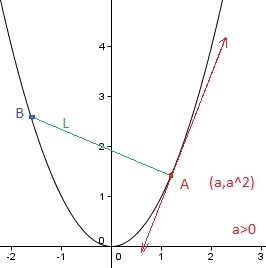# Dancing On a Parabola

Calculus Level 2Suppose that two people, A and B, walk along the parabola $y=x^2$ in such a way that the line segment $L$ between them is always perpendicular to the line tangent to the parabola at A's position $(a,a^2)$ with $a > 0$. If B's position is $(b,b^2)$, what value of $b$ minimizes $L$?

×

Problem Loading...

Note Loading...

Set Loading...